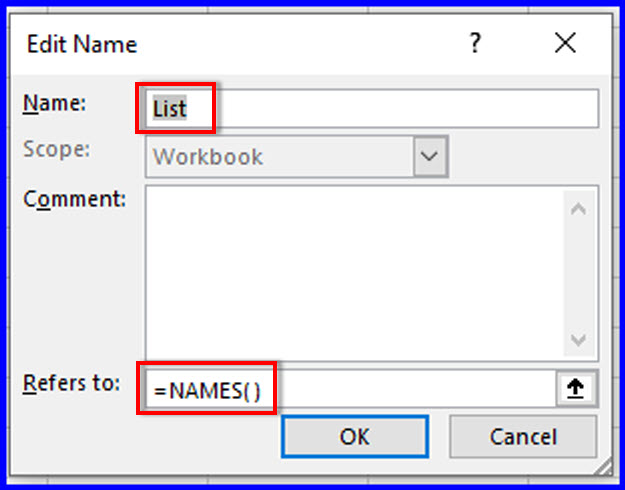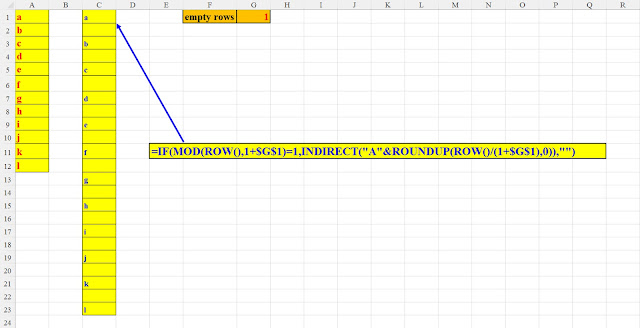## יום שני, 22 באוגוסט 2022

### Excel – how do we find the Column Number?

Excel – how do we find the Column Number?

Every Excel user knows that an address of  a cell comprises of a column (Alphabetic) and a row (numeric).
But the column name represents a number:
Column A is the first, therefore its number is: 1,
Column B is the second, therefore its number is: 2 and so on.
But have you ever wondered: How do we find the column NUMBER from its name?
What column number is: ABC, QZD, FFF, DAT, RRR, X etc.???

What's the solution?

There are, actually, two solutions:

The first, very simple and straightforward:

For example, if you want to find the number of column AAA,

Just type the following formula:
=COLUMNS(A:AAA)

And the result: 703

Pic 1: Find the column's number – method 1

The second is a formula that I've developed, which yields the same result.

A short explanation of my formula:
The English alphabet has 26 letters, from A to Z.
So, for example, if we're on column Z, then we're on column no.26.

If the column name consists of 2 letters (for example: AA), then we need to multiply the first letter A (representing 1) by 26 and then add 1 (the second A).

If the column name consists of 3 letters (for example: AAA), then we need to multiply the first letter A (representing 1) by 26^2, and then:
add 1 (the second A) multiplied by 26 and finally:
add the third A (which is 1),

So: AAA = 1*26^2 + 1*26^1 + 1*26^0 = 676+ 26 + 1 = 703

And why do we subtract 64?

Because the ASCII codes of the English uppercase letters begin with: 65 (=A).

In order to find the number by the letter, we need to subtract 64.

A (=65) becomes 1, B (=66) becomes 2….. and Z (=90) becomes 26.

That's all.

Pic 2: Find the column's number – method 2

Hope you enjoyed this post.

Of course, you could change the columns from alphabetic to numeric,

But then, you won't be able to know what was the original columns' names….

Converting the reference style to R1C1, thus:# Three methods to display a workbook's named ranges

Sometimes, when your workbook has many named ranges, you'd like to have them as a list, in a separate worksheet.

There are at least three methods to accomplish this task:

1.   The shortest method

1)    Press: the F3 key.
the following pane appears:

Pic 1: Press F3

2)    Now, press: ALT+L (or just click the Paste List button)
All the named ranges will be pasted into the worksheet.

2.   Using a defined name in the Name Manager

1)      Press: CTRL+F3 to get to the Name Manager

2)    Click: New (or press: ALT+n) to define a new name

3)    In the Name textbox enter: List (or any name you like)

4)    In the Refers to textbox enter: =NAMES()Pic 2: define a named range that refers to the NAMES() command

5)    In an empty cell of the worksheet, type:
=TRANSPOSE(List)

All the workbook names will be pasted into the worksheet.

3.   VBA

Add the following code to the VBA editor and run it:

Pic 3: Add this code to the VBA editor and run it

After running the code you'll see the list of all the workbook's names in a new worksheet.

## יום שלישי, 16 באוגוסט 2022

### How many active months

How many active months

A client of mine came to me with this problem:

Once a year he gets a yearly activity of items. Since the data is sent from a legacy system, Each item's activity is represented by a 24-character string which contains only digits. Each two digits represent a month, so we have 12 consecutive months in each data item,
as can be seen in the picture (the data are painted for better visibility)

Pic1: the raw data – each 2 digits represent a month

The problem:
We want to know, from the year, how many months yielded an activity.
If the 2-digits are "00", then there was no activity in that month.
Otherwise, that month had an activity and should be counted.

The solution can be achieved with one formula:

Pic 2: Solution in one formula

The formula can be better understood by splitting it into two parts:

Part 1:
create an array of 12 horizontal cells, each cell for a month.

This can easily be accomplished with my favourite function: SEQUENCE.

The multiplication by 1 converts the text digits into "real" numbers.

Pic 3: Part 1 of the solution – creating a numeric array of 12 months

Part 2:

Using any method of conditional counting, to count only non-zero numbers in the array. This can be done in several ways.

For example:

1)   Using the same formula of Part 1 and wrapping it with: SUM(IF(…..),1,0)

Pic 4: Part 2 of the solution – the same formula

2)   COUNTIF with the spilled array

Pic 5: Part 2 of the solution – two formulae

## יום ראשון, 14 באוגוסט 2022

### Add any number of empty rows to a range

Add any number of empty rows to a range

This is a sequel to my original post:
Add alternate empty rows to a range – the coolest method

After publishing it, I thought:

Why not create a generic solution?
Why add only 1 row? Why not 2 or 3 or more?
So I came up with a Parameterized solution:

Put the desired number of empty rows in one formula.

And lo and behold, you have it, like magic.

Attached are three pictures, demonstrating my generic solution:
The first, only one empty row

The second, two empty rows

The third, three empty rows.

Enjoy 😊Pic 1 - Add one empty row

Pic 2 - Add two empty rows

Pic 3 - Add three empty rows

## יום שבת, 13 באוגוסט 2022

### Add alternate empty rows to a range – the coolest method

Add alternate empty rows to a range – the coolest method

Suppose you have a range of consecutive data in column A and you want to add alternate empty rows between the range's cells.

How can this be done in the shortest, most elegant way?

Well, there are several methods to accomplish this task.

But they are all time-consuming because they demand several steps.

Here's the coolest method, that uses only one formula:

## יום שני, 1 באוגוסט 2022

### Shortest method to navigate to a worksheet

Shortest method to navigate to a worksheet

Your workbook is huge. It has dozens of sheets. After working in a certain sheet you need to return to the Master sheet/Index sheet or whatever name you call it.

You could, of course, add a hyperlink to the master sheet from each and every sheet but that, of course, isn't practical.

On the other hand, the "classical" method (as shown in the next two pictures) is also a cumbersome and time-consuming two-phase process.

Old method 1: right-click the navigation icon < to display the worksheets' list

Old method 2: scroll through the list. When you reach the sheet press OK

So what's the best solution?

Creating a named range and using it with one click
😊

1.   Press CTRL+F3 to go to the Name Manager

CTRL+F3: Opening the Name Manager

2.   Press Alt+N (or simply click the New… button) to define a new name

3.   In the New Name pane that opens, click: O (for One) in the name. Of course, you can choose any name. I chose the shortest 'cause I'm lazy 😊

4.     In the Refers to type: =INDEX!\$A\$1 (or: Sheet1!\$A\$1 or whatever the name of your master sheet is) and press: OK

Defining the shortcut to the INDEX worksheet

Now, from any worksheet, in order to navigate to the INDEX sheet, just:
1. CTRL+G (or F5) to open the go to pane

2. Type: O

3. Press: OK

Fastest navigation to the target sheet

If you're nimble enough, you can do it in less than 200 milliseconds !!!!

Do you have a faster way?

### תוכנה שפיתחתי ב"אקסל" – מתרגמת סכומים למילים בעברית

תוכנה שפיתחתי ב"אקסל" – מתרגמת סכומים למילים בעברית

רוצים לתרגם סכומים למילים בעברית?

תוכנה שפיתחתי ב"אקסל" מתרגמת סכומים לעברית:

משקל אחד ועד 999 מיליארד.

צפו בסרטון המצורף.

מעוניינים?

צרו אתי קשר בנייד המופיע בסרטון

אה, עיקר שכחתי: